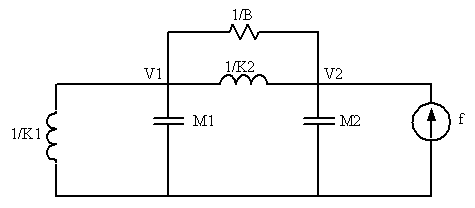Find the Differential Equation Relating Input Voltage and Output Voltage

Hey all,

I'm stuck on a dynamic systems question, it's attached as a jpeg

I started off by writing nodal equations for each node:

Node 1: 1/R1(ei-eA)=C1D(eA-eo)+1/R2(eA-eB)

Node 2:1/R2(eA-eB)=C2D(eB)

I know that I have to isolate for ei and eo but I'm really confused with the D^2 that's in the answer. Any help is greatly appreciated! :)

Attachments

•dynamic systems question.jpg
19 KB · Views: 759

gneill
Mentor
Write the node equations in the state space domain (Laplace); The impedance of a capacitor is given by 1/(s*C). The state variable 's' translates to the differential operator D → ##\frac{d}{dt}## in the time domain.

I know that I have to isolate for ei and eo but I'm really confused with the D^2 that's in the answer.

It's the second derivative DDy -> D2y

For example, if you took the derivative of your second node equation:

1/R2(eA-eB) = C2D(eB)
D(1/R2(eA-eB)) = D(C2D(eB))
1/R2 D(eA-eB) = C2 D2(eB)

Ohhhh okay that makes sense. Took a while to wrap my head around it but I got the right answer in the end. Thanks! :)

While I'm at it, I'm having trouble with another question like the one above, but this time it's a mechanical system. It's the jpeg below.

I started off with the writing equations for each mass

Mass 1: K1x1 + DB(x) + k2(x1-x2) = 0

Mass 2: m2D^2x = DB(x1-x2) + k2(x1-x2)

First off, can someone confirm that those equations are right?

After that, I'm supposed to sub in the given values and get everything in terms of fa(t) and x1, but I feel like my equations are wrong because it's not working out.

Thanks for looking!

Attachments

gneill
Mentor
While I'm at it, I'm having trouble with another question like the one above, but this time it's a mechanical system. It's the jpeg below.

I started off with the writing equations for each mass

Mass 1: K1x1 + DB(x) + k2(x1-x2) = 0

Mass 2: m2D^2x = DB(x1-x2) + k2(x1-x2)

First off, can someone confirm that those equations are right?

After that, I'm supposed to sub in the given values and get everything in terms of fa(t) and x1, but I feel like my equations are wrong because it's not working out.

Thanks for looking!

You've used variables x, x1, and x2 in your equations. I can see x1 and x2 indicated on your diagram, but what is x?

The method that I usually prefer for these sorts of problems is to convert the mechanical system into its equivalent electrical analog. Then I have a available all the analytical apparatus for electrical circuits (including circuit simulators). Your problem "translates" into:Note that potentials at V1 and V2 will represent the velocities of the masses. In the Laplace domain, position x1(s) can be found by integrating velocity V1(s). Integration is trivially accomplished by dividing by the Laplace state variable: x1(s) = V1(s)/s.